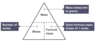Reaction Quantities Flashcards Preview

CfE National 5 Chemistry Unit 1 > Reaction Quantities > Flashcards

Flashcards in Reaction Quantities Deck (14):
1

2

3

4

To calculate the mass of a number of moles, or calculate the number of moles in a certain mass, a formula triangle can be used.Describe it.5

6

7

If the number of moles of solute and the volume of solvent used is known, the concentration of the solution can be calculated.Describe the formula triangle.8

9

10

11

12

13

14